Home | Study Links | Rhymes |

## Planet DiametersPlanets, ratios and the Pythagorean 3-4-5 drawing where the Moon fits the square of 3,
and the Earth diameter ratio is 11 (4+3+4). One can get quite accurate planet diameters by
using the Pythagorean drawing! Furthermore, the two circles just beyond Earth's physical circle
represent our atmospheric layers. Measured here as 65 miles +/- 100 km for the first layers and the
next one at 254 miles +/- 400 km. (ionosphere)

9 Planet diameters: Planet diameter / 720 = Ratio number. Example, Earth 7920 / 720 = 11
1. Mercury --= 3054-----mi / 720 = 4.24
2. Venus-----= 7517-----mi / 720 = 10.44
3. Earth -----= 7920-----mi / 720 = 11
---Moon -----= 2160 ----mi / 720 = 3
4. Mars ------= 4213-----mi / 720 = 5.85
5. Jupiter----= 87120---mi / 720 = 121 (Jupiter = 11 Earth diameters)
6. Saturn-----= 73296---mi / 720 = 101.8 (Saturn = 24 Mercury diameters)
7. Uranus---- = 31680---mi / 720 = 44 (Uranus = 4 Earth diameters)
8. Neptune--= 30600---mi / 720 = 42.4 (Neptune = 10 Mercury diameters)
9. Pluto ------= 1440----mi / 720 = 2____
Total nine planets ----------------= 342.72A possible link between the Antikythera Mechanism and Planet size ratio's.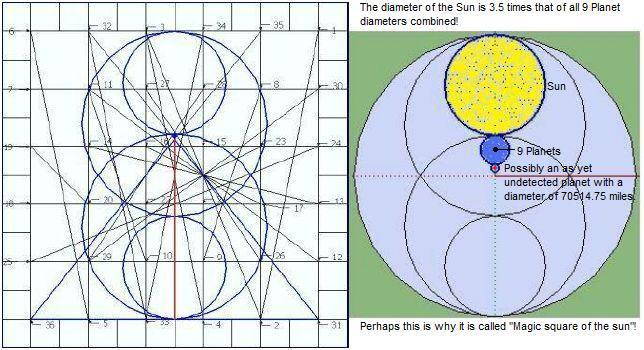Based on the magic square of the sun design and the previously established 9 Planet ratio
number 343, it suggests that there exists another large, and as yet undiscovered planet in
our solar system, with a diameter of 70514.75 miles or so! Ratio number 97.94 perhaps?)

Sun diameter measurements:
A Sun diam. of 864000 mi / 720 = 1200.0 / 3.5 = 342.857142857...
A Sun diam. of 864360 mi / 720 = 1200.5 / 3.5 = 343 (7x7x7)

And a few more observations:
Sun ratio 1200.0 = 864000 (diameter) divided by 2000 is 432, and 4322= A lightspeed of 186624 mps
Sun ratio 1200.5 = 864360 (1200.5 sits between a prime and a non-prime number, 1201 and 1200.)
1200.5 x 2 = 2401 and interestingly 72= 49, and 492= 2401 or 7 x 7 = 49 x 7 = 343 x 7 = 2401
A Sun diam of 864360 miles divided by the most distant planet Pluto diam 1440 = 600.25 x 4 = 2401
and 864360 / 2401 = 360

Earth's atmospheric layers:
Sandwich the ionosphere between 65 mi(+/- 100 km) and 254 mi(+/- 400 km).
65 x 2 = 130 + 7920 = 8050 miles / 720 = 11.18...2 = 125
253.69...x 2 = 507.38...+ 7920= 8427.38...miles / 720 = 11.70...2= 137
8427.38...mi= 2 Mars diameters (2 x 4213.69 mi.)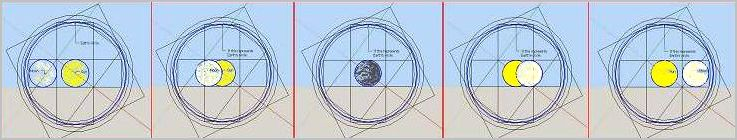Another example of accuracy concerns the two great lights in the sky and the fact that when
viewed from Earth they appear to have identical diameters. The Sun is 400 times wider than
the Moon (400 x 2160), and the distance between Earth and Sun is 400 times the distance
between Earth and Moon, which is a 400 to 1 ratio!

God speaking to Job: "Where wast thou when I laid the foundations of the earth? declare,
if thou hast understanding. 5 Who hath laid the measures thereof, if thou knowest? or who
hath stretched the line upon it? 6 Whereupon are the foundations thereof fastened? or who
laid the corner stone thereof; 7 When the morning stars sang together, and all the sons of
God shouted for joy?"Job 38: 4 Kjv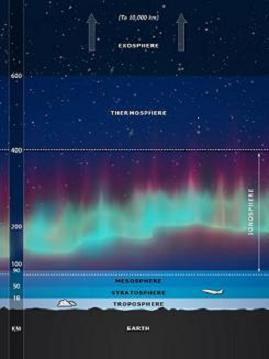The ionosphere (layer between 100 and 400 km) is the area where the Northern and Southern
Auroras occur, and as the planet ratio drawing clearly shows, it all shows perfect design like a
clock or a precision timepiece!The 3° difference between this Axial tilt method @ 26.565° and Earth's present-day tilt @ 23.5°
The arms of this cross multiplied by 1.25 equals it's length.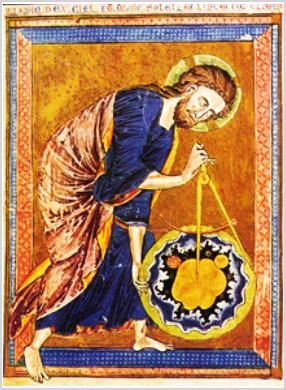"In the beginning God created the heaven and the earth." (Genesis 1:1) The gematria ( sum,
using numerical values of its letters ) of these first 7 words of the book of Genesis is 2701

"In the beginning was the Word and the Word was with God, and the Word was God. 2 The same
was in the beginning with God. 3 All things were made by him; and without him was not anything

"Who hath measured the waters in the hollow of his hand, and meted out heaven with the
span, and comprehended the dust of the earth in a measure, and weighed the mountains in
scales, and the hills in a balance?" (Isaiah 40:12)

Click on the image for its origin.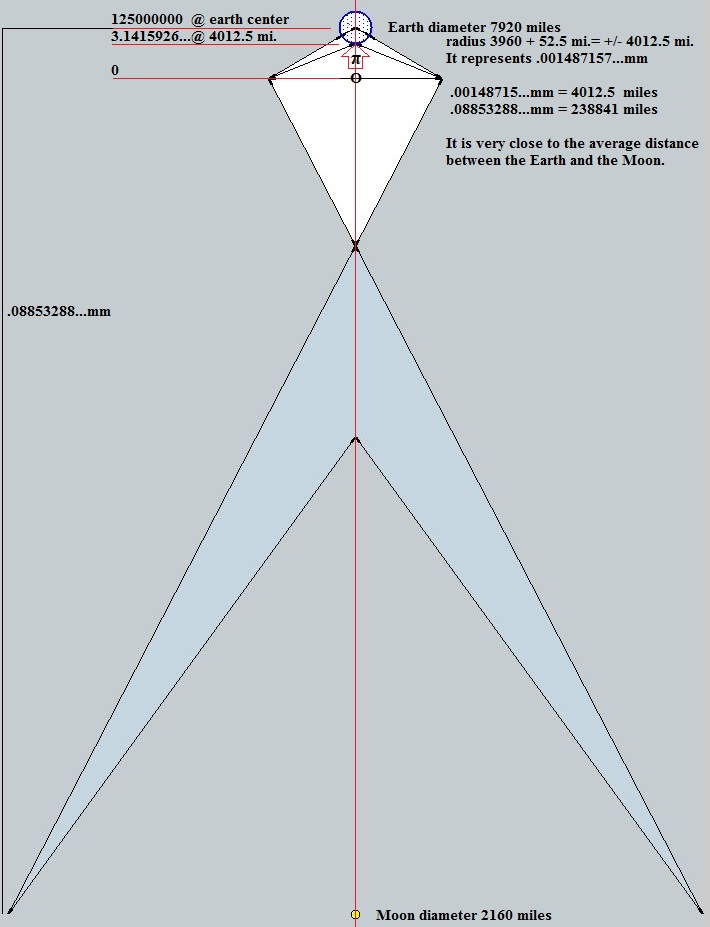Lastly, a few ideas on Spirals, Circles, and the Numbers 3-6-9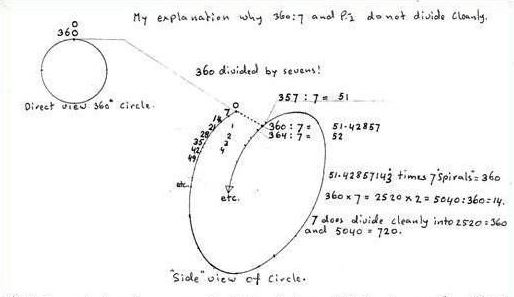Spirals to form a Torus or donut shape, 21, 42, 63, etc.
Bible verse: "That which hath been is now; and that which is to be hath already been" Ecc 3:15
The past is now; and future is already past. A circular description perhaps?

360 / 7 and .14 28 57... x 360= 51.42857142857... repeats 14 28 57. And 360 / .14 28 57...= 2520= 7 x 360

.14 28 57... x 1= .14 28 57...
.14 28 57... x 2= .28 57 14...
.14 28 57... x 3= .42 85 71...
.14 28 57... x 4= .57 14 28...
.14 28 57... x 5= .71 42 85...
.14 28 57... x 6= .85 71 42...
.14 28 57... x 7= 1
.14 28 57... x 8= 1.14 28 57...
.14 28 57... x 9= 1.28 57 14...
Etc.
From 21 on, the numbers 3, 6 and 9 are included.
.14 28 57...x 21= 3
.14 28 57...x 42= 6
.14 28 57...x 63= 9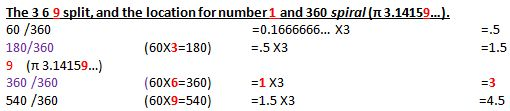Continue with Stonehenge